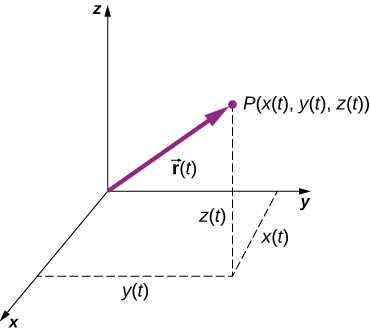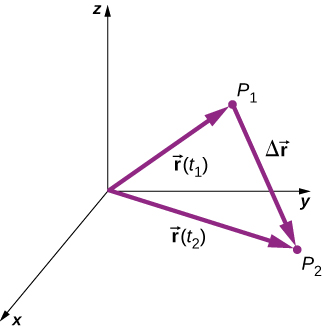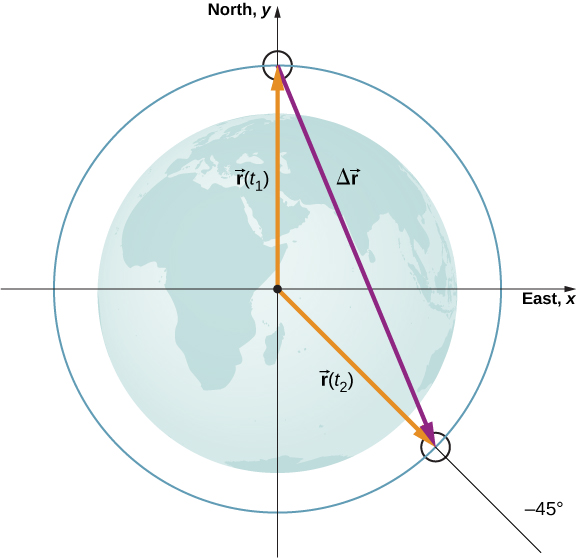# 4.1 Displacement and velocity vectors

 Page 1 / 7
By the end of this section, you will be able to:
• Calculate position vectors in a multidimensional displacement problem.
• Solve for the displacement in two or three dimensions.
• Calculate the velocity vector given the position vector as a function of time.
• Calculate the average velocity in multiple dimensions.

Displacement and velocity in two or three dimensions are straightforward extensions of the one-dimensional definitions. However, now they are vector quantities, so calculations with them have to follow the rules of vector algebra, not scalar algebra.

## Displacement vector

To describe motion in two and three dimensions, we must first establish a coordinate system and a convention for the axes. We generally use the coordinates x , y , and z to locate a particle at point P ( x , y , z ) in three dimensions. If the particle is moving, the variables x , y , and z are functions of time ( t ):

$x=x\left(t\right)\phantom{\rule{1em}{0ex}}y=y\left(t\right)\phantom{\rule{1em}{0ex}}z=z\left(t\right).$

The position vector    from the origin of the coordinate system to point P is $\stackrel{\to }{r}\left(t\right).$ In unit vector notation, introduced in Coordinate Systems and Components of a Vector , $\stackrel{\to }{r}\left(t\right)$ is

$\stackrel{\to }{r}\left(t\right)=x\left(t\right)\stackrel{^}{i}+y\left(t\right)\stackrel{^}{j}+z\left(t\right)\stackrel{^}{k}.$

[link] shows the coordinate system and the vector to point P , where a particle could be located at a particular time t . Note the orientation of the x , y , and z axes. This orientation is called a right-handed coordinate system ( Coordinate Systems and Components of a Vector ) and it is used throughout the chapter.A three-dimensional coordinate system with a particle at position P ( x ( t ), y ( t ), z ( t )).

With our definition of the position of a particle in three-dimensional space, we can formulate the three-dimensional displacement. [link] shows a particle at time ${t}_{1}$ located at ${P}_{1}$ with position vector $\stackrel{\to }{r}\left({t}_{1}\right).$ At a later time ${t}_{2},$ the particle is located at ${P}_{2}$ with position vector $\stackrel{\to }{r}\left({t}_{2}\right)$ . The displacement vector     $\text{Δ}\stackrel{\to }{r}$ is found by subtracting $\stackrel{\to }{r}\left({t}_{1}\right)$ from $\stackrel{\to }{r}\left({t}_{2}\right)\text{ }:$

$\text{Δ}\stackrel{\to }{r}=\stackrel{\to }{r}\left({t}_{2}\right)-\stackrel{\to }{r}\left({t}_{1}\right).$

Vector addition is discussed in Vectors . Note that this is the same operation we did in one dimension, but now the vectors are in three-dimensional space.The displacement Δ r → = r → ( t 2 ) − r → ( t 1 ) is the vector from P 1 to P 2 .

The following examples illustrate the concept of displacement in multiple dimensions.

## Polar orbiting satellite

A satellite is in a circular polar orbit around Earth at an altitude of 400 km—meaning, it passes directly overhead at the North and South Poles. What is the magnitude and direction of the displacement vector from when it is directly over the North Pole to when it is at $-45\text{°}$ latitude?

## Strategy

We make a picture of the problem to visualize the solution graphically. This will aid in our understanding of the displacement. We then use unit vectors to solve for the displacement.

## Solution

[link] shows the surface of Earth and a circle that represents the orbit of the satellite. Although satellites are moving in three-dimensional space, they follow trajectories of ellipses, which can be graphed in two dimensions. The position vectors are drawn from the center of Earth, which we take to be the origin of the coordinate system, with the y -axis as north and the x -axis as east. The vector between them is the displacement of the satellite. We take the radius of Earth as 6370 km, so the length of each position vector is 6770 km.Two position vectors are drawn from the center of Earth, which is the origin of the coordinate system, with the y -axis as north and the x -axis as east. The vector between them is the displacement of the satellite.

what is vector
A quantity having both magnitude and direction
Zubair
How to calculate for overall displacement
Well, take Final Position-Starting position
Grant
what is velocity
speed per unit time is called velocity. it is a vector quantity
Mukulika
velocity is distances overall time taking,it is a vector quantity, the units is metre per second.
Samuel
frequency is rate at which something happens or is repeated. it is a vector quantity
mawuo
what is the difference between resultant force and net force
net force is when you add forces numerically I.e. the total sum of all positive and negative or balanced and unbalanced forces. resultant force is a single vector which is the combination or addition of all x and y axes vector component forces in a system.
emmanuel
thanks
Ogali
resultant force is applied to hold or put together an object moving at the wrong direction. in other words it repairs.
Andrew
Damping is provided by tuning the turbulence levels in the moving water using baffles.How it happens? Give me a labelled diagram of it.
A 10kg ball travelling at 4meter per second collides elastically in a head-on collision with a 2kg ball.What are (a)the velocities and (b)the total momentum of the balls after collision?
a)v1 8/3s&v2 20/3s. b)in elastic collision total momentum is conserved.
Bala
multiply both weight which is 10*2 divided by the time give 4. and our answer will be 5.
Andrew
The displacement of the air molecules in sound wave is modeled with the wave function s(x,t)=5.00nmcos(91.54m−1x−3.14×104s−1t)s(x,t)=5.00nmcos(91.54m−1x−3.14×104s−1t) . (a) What is the wave speed of the sound wave? (b) What is the maximum speed of the air molecules as they oscillate in simple harmon
the question is wrong. if you need assistance with displacement I can help out.
Andrew
practical 1st year physics
huh
Luminous
allot of practicals, be specific with your topic and we can discuss.
Andrew
Whats the formular for newton law of motion
f=ma
F=m×a Where F=force M=mass of a body of an object a=acceleration due to gravity
Abubakar
what is speed
distance travelled per unit of time is speed.
distance travelled in a particular direction it is.
Andrew
Speed is define as the distance move per unit time. Mathematically is given as Speed = distance/time Speed = s/t
Abubakar
speed is a vector quantity. It is defined distance per unit time.It's unit in c.g.s cm/s and in S.I. m/s.It’s dimension is LT^-1
Mukulika
formula for velocity
v=ms^-1 velocity=distance time
Cleophas
(p-a/v)(v-b)=nrt what is the dimension of a
Amraketa
velocity= displacement time
Gold
Velocity = speed/time
Abubakar
what are evasive medical diagnosis?
If the block is displaced to a position y , the net force becomes Fnet=k(y−y0)−mg=0Fnet=k(y−y0)−mg=0 . But we found that at the equilibrium position, mg=kΔy=ky0−ky1mg=kΔy=ky0−ky1 . Substituting for the weight in the equation yields. Show me an equation of graph.
Shaina
where are you come from
Lida
samastipur Bihar
carrier
simple harmonic motion defination
how to easily memorize motion equation
Maharam
how speed destrog is uranium
where can we find practice problems?
I'm not well
YAZID
SayedByByByByBy Eric CrawfordByBy